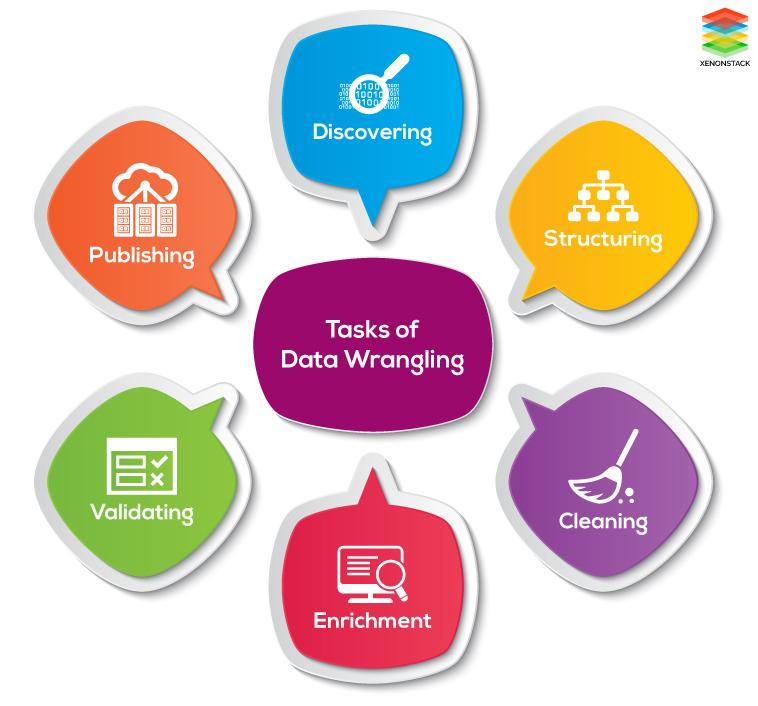+1

# Data Preprocessing and Data Wrangling in Machine Learning and Deep Learning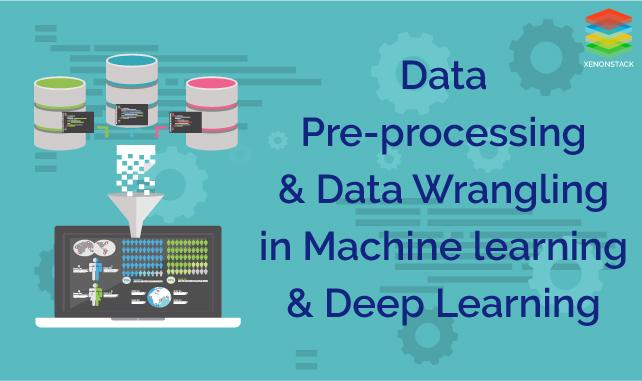# Introduction

Deep learning and Machine learning are becoming more and more important in today's ERP (Enterprise Resource Planning). During the process of building the analytical model using Deep Learning or Machine Learning the data set is collected from various sources such as a file, database, sensors and much more.

But, the collected data cannot be used directly for performing analysis process. Therefore, to solve this problem Data Preparation is done. This includes two techniques that are listed below:

• Data Preprocessing

• Data Wrangling

Data Preparation is an important part of Data Science. It includes two concepts such as Data Cleaning and Feature Engineering. These two are compulsory for achieving better accuracy and performance in the Machine Learning and Deep Learning projects.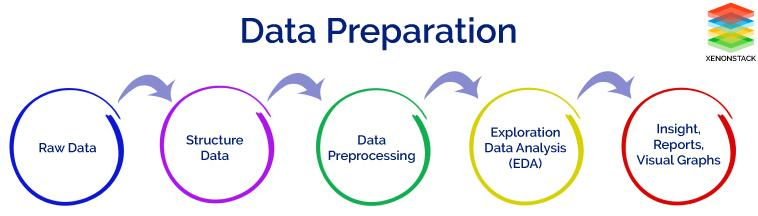# Data Preprocessing

Data Preprocessing is a technique that is used to convert the raw data into a clean data set. In other words, whenever the data is gathered from different sources it is collected in raw format which is not feasible for the analysis.

Therefore, certain steps are executed to convert the data into a tiny clean dataset. This technique is performed before the execution of Iterative Analysis. The set of steps is known as Data Preprocessing. This includes Data Cleaning, Data Integration, Data Transformation and Data Reduction.

# Data Wrangling

Data Wrangling is a technique that is executed at the time of making an interactive model. In other words, it is used to convert the raw data into the format that is convenient for the consumption of data.

This technique is also known as Data Munging. This method also follows certain steps such as after extracting the data from different data sources, sorting of data using certain algorithm is performed, decompose the data into a different structured format and finally store the data into another database.

# Need of Data Preprocessing

For achieving better results from the applied model in Machine Learning and Deep Learning projects the format of the data has to be in a proper manner. Some specified Machine Learning and Deep Learning model need data in a specified format, for example, Random Forest algorithm does not support null values, therefore to execute random forest algorithm null values has to be managed from the original raw data set.

Another aspect is that dataset should be formatted in such a way that more than one Machine Learning and Deep Learning algorithms are executed in one dataset and best out of them is chosen.

# Need of Data Wrangling

Data Wrangling is an important aspect for implementing the model. Therefore, data is converted to the proper feasible format before applying any model intro it. By performing filtering, grouping and selecting appropriate data accuracy and performance of the model could be increased.

Another concept is that when time series data has to be handled every algorithm is executed with different aspects. Therefore Data Wrangling is used to convert the time series data into the required format of the applied model. In simple words, the complex data is converted into a usable format for performing analysis into it.

# Why is Data Preprocessing used?

Data Preprocessing is necessary because of the presence of unformatted real world data. Mostly real world data is composed of -

• Inaccurate data (missing data) - There are many reasons for missing data such as data is not continuously collected, a mistake in data entry, technical problems with biometrics and much more.

• The presence of noisy data (erroneous data and outliers) - The reasons for the presence of noisy data could be a technological problem of gadget that gathers data, a human mistake during data entry and much more.

• Inconsistent data - The presence of inconsistencies is due to the reasons such that existence of duplication within data, human data entry, containing mistakes in codes or names i.e. violation of data constraints and much more.

Therefore, to handle raw data, Data Preprocessing is performed.

# Why is Data Wrangling used?

Data Wrangling is used to handle the issue of Data Leakage while implementing Machine Learning and Deep Learning. First of all, we have to understand what is Data Leakage?

### What is Data Leakage in Machine Learning/Deep Learning?

Data Leakage is responsible for the cause of invalid Machine Learning/Deep Learning model due to the over optimization of the applied model.

Data Leakage is the term used when the data from outside i.e. not part of training dataset is used for the learning process of the model. This additional learning of information by the applied model will disapprove the computed estimated performance of the model.

For example when we want to use the specific feature for performing Predictive Analysis but that specific feature is not present at the time of training of dataset then data leakage will be introduced within the model.

Data Leakage can be demonstrated in many ways that are given below:

• Leakage of data from test dataset to training dataset.

• Leakage of computed correct prediction to the training dataset.

• Leakage of future data into the past data.

• Usage of data outside the scope of applied algorithm

In general, the leakage of data is observed from two main sources of Machine Learning/Deep Learning algorithms such as feature attributes (variables) and training dataset.

### How to check the presence of Data Leakage within the applied model?

Data Leakage is observed at the time of usage of complex datasets. They are described below:

• At the time of dividing time series dataset into training and test, the dataset is a complex problem.

• Implementation of sampling in a graphical problem is a complex task.

• Storage of analog observations in the form of audios and images in separate files having a defined size and timestamp.

# How is Data Preprocessing performed?

Data Preprocessing is performed to remove the cause of unformatted real world data which are discussed above.

First of all, let's discuss how missing data can be handled. There are three different steps that can be executed which are given below:

• Ignoring the missing record - It is the simplest and effective method for handling the missing data. But, this method should not be performed at the time when the number of missing values are huge or when the pattern of data is related to the unrecognized basic root of the cause of statement problem.

• Filling the missing values manually - This is one of the best-chosen methods. But there is one limitation that when there is large dataset and missing values are large then, this method is not efficient as it becomes a time-consuming task.

• Filling using computed values - The missing values can also be filled by computing mean, mode or median of the observed given values. Another method could be the predictive values that are computed by using any Machine Learning or Deep Learning algorithm. But one drawback of this method is that it can generate bias within the data as the computed values are not accurate with respect to the observed values.

Let's move further and discuss how we can deal with the noisy data. The methods that can be followed are given below:

• Binning method - In this method sorting of data is performed with respect to the values of the neighborhood. This method is also known as local smoothing.

• Clustering method - In the approach, the outliers may be detected by grouping the similar data in the same group i.e. in the same cluster.

• Machine Learning - A Machine Learning algorithm can be executed for smoothing of data. For example, regression algorithm can be used for smoothing of data using a specified linear function.

• Removing manually - The noisy data can be removed manually by the human being but it is a time-consuming process so mostly this method is not given priority.

To deal with the inconsistent data manually the data is managed using external references and knowledge engineering tools like knowledge engineering process.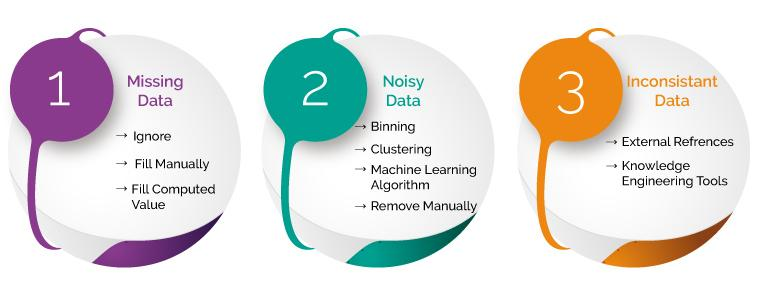# How is Data Wrangling performed?

Data Wrangling is performed to minimize the effect of Data Leakage while executing the model. In other words if one consider the complete dataset for normalization and standardization, then the cross-validation is performed for the estimation of the performance of the model leads to the beginning of data leakage.

Another problem is also observed that the test model is also included for feature selection while executing each fold of cross-validation which further generates bias during performance analysis.

The effect of Data Leakage could be minimized by recalculating for the required Data Preparation during the cross-validation process that includes feature selection, outliers detection, and removal, projection methods, scaling of selected features and much more.

Another solution is that dividing the complete dataset into training dataset that is used to train the model and validation dataset which is used to evaluate the performance and accuracy of the applied model.

But, the selection of the model is made by looking at the results of test dataset in the cross validation process. This conclusion will not always be true as the sample of test dataset could vary and the performance of different models are evaluated for the particular type of test dataset. Therefore, while selecting best model test error is overfitting.

To solve this problem, the variance of the test error is determined by using different samples of test dataset. In this way, the best suitable model is chosen.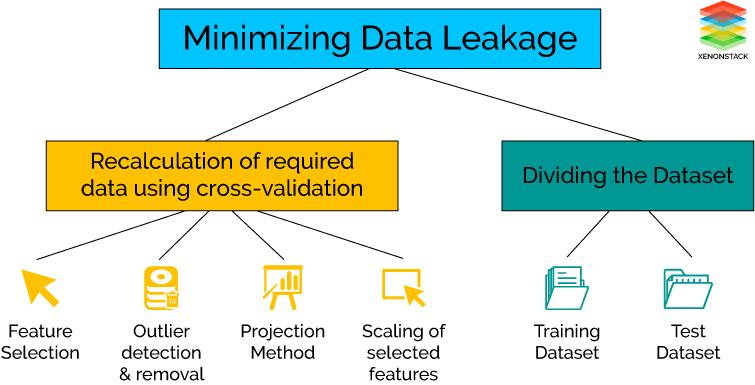# Difference Between Data Preprocessing and Data Wrangling

Data Preprocessing is performed before Data Wrangling. In this case, Data Preprocessing data is prepared exactly after receiving the data from the data source. In this initial transformations, data cleaning or any aggregation of data is performed. It is executed once.

For example, we have a data where one attribute have three variables and we have to convert them into three attributes and delete the special characters from them. This is the concept that is performed before applying any iterative model and will be executed once in the project.

On the other hand, Data Wrangling is performed during the iterative analysis and model building. This concept at the time of feature engineering. The conceptual view of the dataset changes as different models is applied to achieve good analytic model.

For example, we have data containing 30 attributes where two attributes are used to compute another attribute and that computed feature is used for further analysis. In this way, the data could be changed according to the requirement of the applied model.

Different steps are involved for Data Preprocessing. These steps are described below:

• Data Cleaning - This is the first step which is implemented in Data Preprocessing. In this step, the main focus is on handling missing data, noisy data, detection, and removal of outliers, minimizing duplication and computed biases within the data.

• Data Integration - This process is used when data is gathered from various data sources and data is combined together to form consistent data. This consistent data after performing data cleaning is used for analysis.

• Data Transformation - This step is used to convert the raw data into a specified format according to the need of the model. The options used for transformation of data are given below:

• Normalization - In this method, numerical data is converted into specified range i.e. between 0 and 1 so that scaling of data can be performed.

• Aggregation - The concept can be derived from the word itself, this method is used to combine the features into one. For example combining two categories can be used to form a new category.

• Generalization - In this case, lower level attributes are converted into a higher level.

• Data Reduction - After the transformation and scaling of data duplication i.e. redundancy within the data is removed and organize the data in an efficient manner.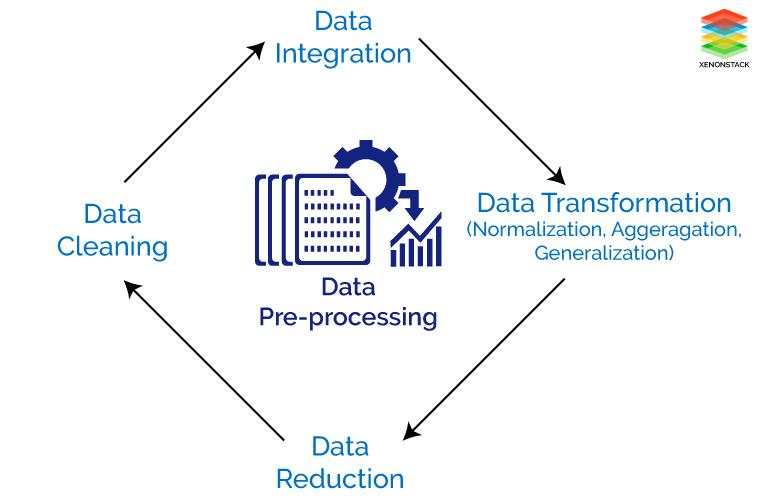The tasks of Data wrangling are described below -

• Discovering - Firstly, data should be understood thoroughly and examine which approach will best suit. For example: if have a weather data when we examine the data it is observed that data is from one area and so main focus is on determining patterns.

• Structuring - As the data is gathered from different sources, the data will be present in different shapes and sizes. Therefore, there is a need of structuring the data in proper format.

• Cleaning - Cleaning or removing of data should be performed that can degrade the performance of analysis.

• Enrichment - Extract new features or data from the given dataset in order to optimize the performance of the applied model.

• Validating - This approach is used for improving the quality of data and consistency rules so that transformations that are applied to the data could be verified.

• Publishing - After completing the steps of Data Wrangling, the steps can be documented so that similar steps can be performed for similar kind of data to save time.